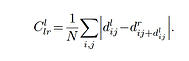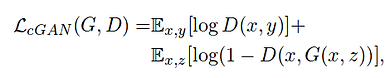# Unsupervised Monocular Depth Estimation

## Motivation and Objective

High fidelity disparity maps are hard to generate, often requiring expensive time of flight sensors or simulated data. In case of LIDARs, the depth data generated is  sparse. Techniques like SGBM and graph cuts often run in order of seconds and produce noisey results. This was class project wherein I designed a CNN architecture that learns to predict depth, as shown in Fig. 1, in an unsupervised fashion, just using left and right stereo pairs.Fig. 1 (a) The image of Sorghum Stalks.

Fig. 1 (b) Corresponding generated Disparity Map.

## Unsupervised Monocular Depth Estimation

The CNN architecture shown in Fig. 2 learns to produce depth maps corresponding to left and right stereo images, given the left stereo image. A set of convolutional layers take the left image as input and then an up-conv layer learns to produce the depth maps corresponding to the left and right frames.Fig. 2 The network learns to predict left and right Disparity Maps, given left image as input.

These disparity maps are then used to reconstruct the images, by sampling the original images according to the corresponding disparity maps. This is done at various levels in the Conv-Net, as shown in Fig.3.

Fig. 3 This figure shows the reconstructed images after sampling the pixels form the original images according to their respective disparity maps

The network is trained to produce good disparity maps by minimizing the following losses:

1. Depth consistency between the left and right disparity map, shown in eqn.(1)

2. Image reconstruction loss, on the image interpolated from estimated depth map. This is shown by eqn. (2)

3. Disparity smoothness loss, that enforces smooth disparity. This is shown in eqn.(3)(1)

(2)(3)

• GANs are generative CNNs that have a generator CNN that learns to reproduce the data from training distribution and a Discriminator, that learns to discriminate between real and reproduced data.

• C-GANs condition their output on data from input domain and produce data that belongs to output domain. The Discriminator learns to discriminate between real output domain image and reproduced output domain image.

• Generator and Discriminator play an adversarial game wherein, the system reduces the loss as depicted by eqn. 4Fig.4. The architecture for a Conditional Generative Network. Given the original image in domain A(un-colored), the Generator tries to generate the same image but in domain B(colored). The discriminator is trained to detect weather the image it sees is Original image in domain B or reconstructed domain B image.(a)(c)

(b)(d)

• C-GANs condition their output on data from input domain and produce data that belongs to output domain. The Discriminator learns to discriminate between real output domain image and reproduced output domain image.

• Here, fig. 5 (a and b) show the input domain A (grey-scale image) and  generate output domain B (color) image.

• And, since no project is ever completed without our lab's agricultural images, fig. 5 (c and d) is another example where the  input image is a colored image of a stalk and the output domain image is an image where the stalk is segmented.

• It can be seen that the c-GAN does a good job in reconstructing the image with stalks segmented form leaves. Architectures like FCN have a had time doing this. This is because the the Generator has is forced to learn more realistic looking images because of Discriminator's loss signal.

Fig. 5 : (a) Sample domain A flower image, (b) sample domain B flower image, (c) sample domain A stalk image and (d) sample domain B stalk image.

• The following loss function gives the error signal for the Generator and the Discriminator.

• Here:

• x : is the input image

• y : is the ground truth image in the output domain

• z : is the noise vector. In this implementation, it is just the drop-out noise.

• G(x,z) : is the generated image given the input image and noise

• The Generator's job is to maximize the loss and the Discriminator's job is to minimize the loss.(4)

Where D tries to minimize the objective and G tries to maximize it.

## Proposed Architecture

• In the proposed architecture, the total loss is a combination of disparity smoothness loss, L1 loss, Left-Right consistency loss and the GAN loss, that comes from the discriminator.

• The idea being, the Discriminator should train to discriminate between real images and images reconstructed by sampling original images with corresponding disparity maps.

• This adversarial training should force the Generator to generate better disparity maps, so that the reconstructed images are more realistic.Fig. 6 This is the proposed architecture. It uses a U-net architecture that learns to predict the dept-maps corresponding to both the stereo images, given the left image. The Discriminator learns to discriminate between the reconstructed image and original image.(5)

(6)

(7)

D

• The Loss function has the following components:

1.            : The that the Discriminator needs to minimize. It is the negative log probability of the prediction of Discriminator for real image and 1- the probability of the fake image being real

2.                     : This loss incentivizes the Generator to generate images such that the Discriminator loss increases by predicting a higher probability of them being real.

3. Gen_Total_Loss  : This is the total loss that the Generator tries to minimize. This is a linear combination of loss for consistency between left and right disparity maps, loss for smoothness of disparity maps and the

Loss

G

Loss_GAN

G

Loss_GAN

## Results And ConclusionNow these are very interesting graphs:

• We can see that  the Discriminator loss goes down  at first. This is because the Generator is generating bad disparity maps. So during the initial phase of training Disparity Smoothness loss and Left-Right Consistency loss help the generator stable depth map representations.

• But as Training progresses, at around 12,000 steps the depth-maps become better. That's when the Discriminator loss starts to increase again. This is when the reconstructions become more realistic and the artifacts due dot depth-map smoothing loss are removed.

• The last figure shows the total loss for the Generator.

(a)(b)

(c)

Fig. 7 (a) The Generator loss, as shown in eqn (6). (b) is the Discriminator loss, eqn.(5) and (c) shows the total loss, as shown by eqn.(7)(a)(c)(b)(d)

• The network was trained with 1500 pairs of stereo images, collected in a Sorghum field. Since, we did not have any ground truth data for depth-maps, the evaluation was done over synthetically generated images in the MPI Sintel data-set. The algorithm had a mean absolute error of 9.28% from the ground truth disparity map.

• Fig. 8 are depth maps generated for images of Sorghum and MPI SIntel data-set respectively.

Fig.8 (a) and (c) show the sample input images and (b) and (d) show the corresponding generated depth-maps.

Future Work : This was a just a class project with limited resources. In future, I would like to run test the architect over bigger data-set like CityScapes. I would like to delve into techniques to make the training more stable. Possibly use a bigger network, inspired from HD-GAN and do more comprehensive evaluation on open source data-sets